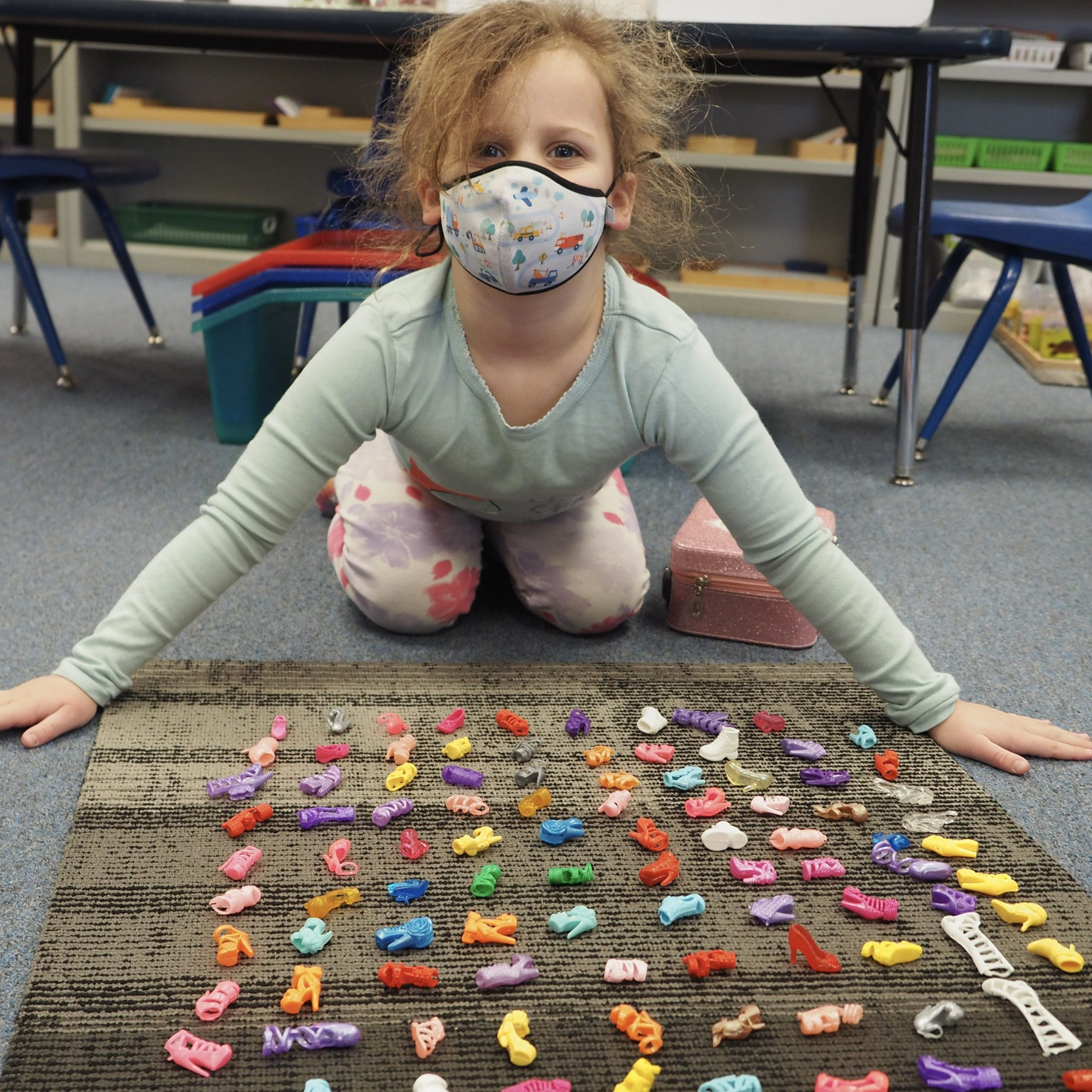מתמטיקה

mathematicsOur math curriculum teaches students to become effective thinkers, problem solvers and mathematical communicators.

Singapore Math teaches for mastery. Each new math skill begins with the use of concrete objects and progresses to pictoral representations and then to abstract algorithms. The goal is mastery of each concept, and students of Singapore Math spend more time solidifying their understanding of a math concept than do students of most other math curricula.In Singapore and around the world, the Singapore Math curriculum begins in first grade. (There is no kindergarten in Singapore.) Our students begin the curriculum with the advantage of having used the Montessori approach to math in Kindergarten. Like Singapore Math, Montessori uses materials that move young mathematicians from the concrete to the abstract, moving from one concept to another upon mastery.

In seventh and eighth grades, students transition to year-long study of pre-algebra, algebra and geometry.

STEAM projects in every grade include math components as well, demonstrating a practical application of math in solving design problems.